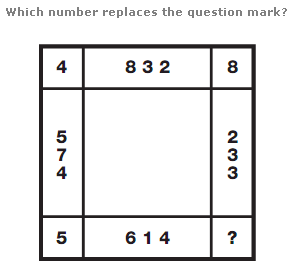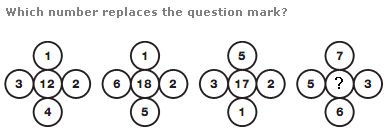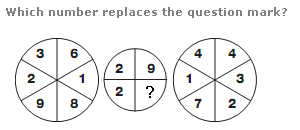# Puzzles - Number puzzles

### Exercise :: Number puzzlesAnswer : 9 Explanation : Take any group of 5 numbers along the side of the square, and their total is always 25.Answer : 29 Explanation : In each diagram, add up the 4 outer numbers then add 2 for the left hand group, 4 for the next, then 6, then 8, and put this result in the central circle.Answer : 1 Explanation : Calculate the sums of the numbers in the left and right hand circles, to give 2 digit answers. Write the left hand answer in the top 2 segments of the central circle, and put the right hand answer in the bottom 2 segments of the central circle.